# Reading the common graphics of univariate statistics

``````## Loading required package: ggplot2
``````

This web page quizzes you on your ability to read the basic graphics
of exploratory data analysis. Just answer the questions below by
eyeballing the graphs. To see how you did, click the “grade” button at
the bottom.

For moderate-sized data sets the stem-and-leaf plot lets us quickly
identify the center, spread and shape of a distribution, as well as
identify quantiles.

Suppose our data set is `x`.

The stem and leaf plot of x is found by:

``````stem(x, scale = 2)
``````
``````##
##   The decimal point is 1 digit(s) to the right of the |
##
##   100 | 18
##   101 | 239
##   102 | 0
##   103 | 7
##   104 |
##   105 | 1
##   106 | 2
##   107 | 2
##   108 | 14
##   109 | 4
##
``````

New problem: What is the maximum value of `x` recorded in the data?

New problem: What is the median value of `x` recorded in the data?

New problem: Is the shape of `x` “long-tailed”

Boxplots allow us to quickly see the center, spread and rough shape of
a distribution in a graphic that invites comparison of many
distributions together (side-by-side boxplots).

``````p <- ggplot(morley, aes(x = factor(Expt), y = Speed))
p + geom_boxplot()
``````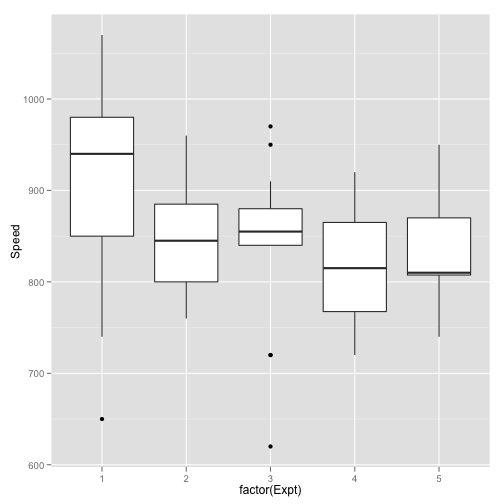The graphic shows a summary of the measured speed of light (in some
scale) for each of 5 experiments recorded in the `morley` data set.

New problem: Which of the 5 experiments have “outliers” as determined by the 1.5 IQR rule?

New problem: Which of the 5 experiments had the largest median value?

New problem: Which of the 5 experiments had the smallest recorded value?

New problem: For experiment 1, the value of the Q3 is more than the maximum value of which experiments?

Histograms allow us to quickly identify the center, spread and shape
of a distribution for arbitrarily large data sets. This is unlike the
stem and leaf plot, an excellent graphic that unfortunately doesn't
scale well to larger sets of numbers.

``````qplot(x, binwidth = diff(range(x))/30)
``````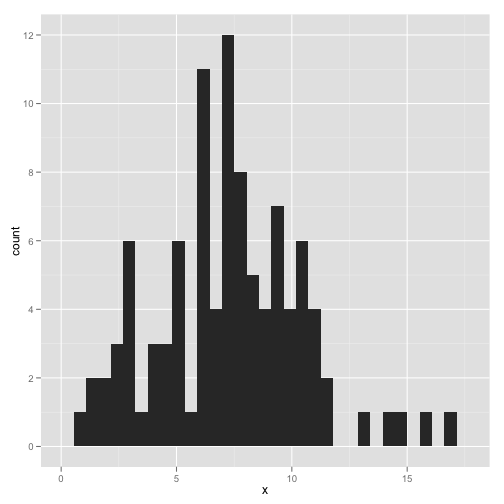Answer the following questions based on the histogram of `x`:

New problem: What is the median value of `x`?

New problem: What is the mean value of `x`?

New problem: Which boxplot best represents `x`:

``````p <- ggplot(d, aes(y = values, x = ind))
p + geom_boxplot()
``````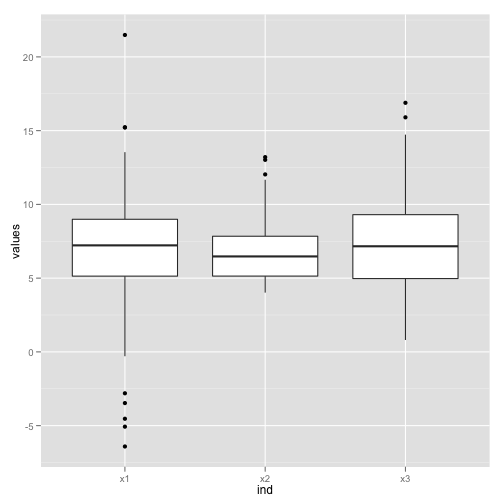A density plot is often seen overlaid a histogram, as in the figure
below. This is a bit redundant, both give a visual estimate of the
parent population of a random sample. The histogram has more ```chart junk```, as Tufte might say, but the density plot is less familiar.

``````p <- ggplot(diamonds, aes(x = carat))
p + geom_histogram(aes(y = ..density..)) + geom_density(alpha = 0.2,
fill = "#FF6666")
``````
``````## stat_bin: binwidth defaulted to range/30. Use 'binwidth = x' to adjust this.
``````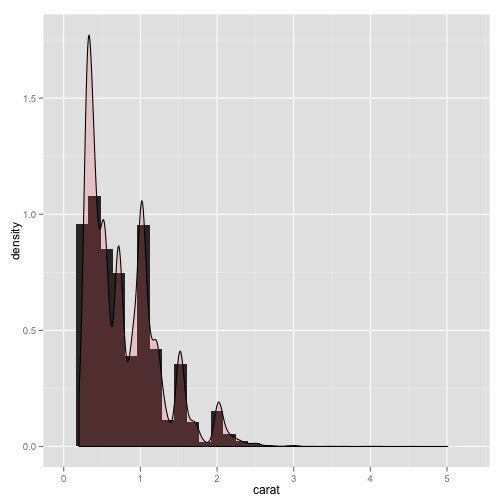New problem: Based on the plot of `carat`, estimate the mean value for this data:

New problem: Based on the shape of the graph, would you say the distribution is

New problem: Based on the shape of the graph, would you say the distribution is

The quantile-quantile plot allows one to compare one distribution
against the other. The two are similar up to changes of scale and
spread if the `qqplot` is essentially straight.

``````df <- data.frame(rivers)
ggplot(df, aes(sample = rivers)) + stat_qq()
``````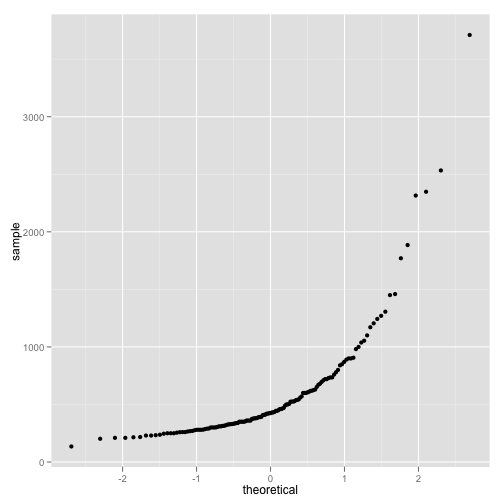New problem: Based on the graphic above, is the `rivers` data approximately normal?